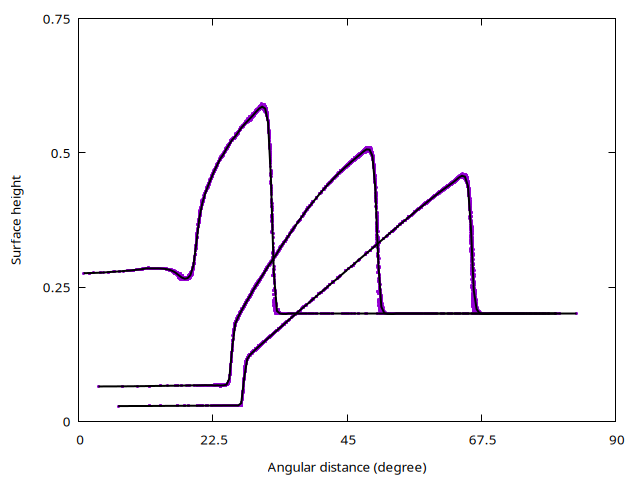# Circular dam break on a sphere

An initial circular cylinder collapses and creates shock and rarefaction waves. The initial condition are radially-symmetric and should remain so. The problem is discretised using longitude-latitude spherical coordinates. Deviations from radial symmetry are a measure of the accuracy of treatment of geometric source terms.

This test case was proposed by Rossmanith et al, 2004, Figures 5 and 6.

#include "spherical.h"
#if ML
# include "layered/hydro.h"
#else
# include "saint-venant.h"
#endif
#include "fractions.h"

int main()
{

The radius of the sphere is unity, the domain is 150 degrees squared, centered on the origin.

  Radius = 1. ;
L0 = 150. ;
X0 = Y0 = -L0/2.;
N = 256;
run();
}

event init (i = 0)
{

To initialise an accurate, sharp initial dam, we use a volume fraction computation. The acos(…) formula is that for the great-circle distance from the origin.

  fraction (h, 0.2 - acos(cos(x*pi/180.)*cos(y*pi/180.)));
double a = 1.8;
foreach()
h[] = 0.2 + a*h[];
}

event masscheck (i++)
{

Mass must be preserved to within machine precision. This is a check of the consistency of the (adaptive) spherical metric.

  stats s = statsf(h);
static double max = -HUGE, min = HUGE;
if (s.sum > max) max = s.sum;
if (s.sum < min) min = s.sum;
assert ((max - min)/(max + min) < 1e-12);
// fprintf (stderr, "%g %g\n", t, (max - min)/(max + min));
}

event profiles (t = 0.3; t += 0.3; t <= 0.9) {

We store the average solution in bins of one degree.

  double xp, yp, np;
for (int i = 0; i < 180; i++)
xp[i] = 0., yp[i] = 0., np[i] = 0.;

foreach() {
printf ("%g %g %g %g %g\n", x, y, u.x[], u.y[], h[]);
double c = cos(x*pi/180.)*cos(y*pi/180.);
double d = atan2(sqrt(1. - c*c),c)*180./pi;
int i = d*2.;
xp[i] += d; yp[i] += h[]; np[i]++;
}

The average profiles.

  char name;
sprintf (name, "prof-%g", t);
FILE * fp = fopen (name, "w");
for (int i = 0; i < 180; i++)
if (np[i] > 0.)
fprintf (fp, "%g %g %g\n", xp[i]/np[i], yp[i]/np[i], np[i]);
fclose (fp);

We compute the RMS error between the grid points and the average profile.

  double sum = 0., n1 = 0.;
foreach() {
double c = cos(x*pi/180.)*cos(y*pi/180.);
double d = atan2(sqrt(1. - c*c),c)*180./pi;
int i = d*2.;
double e = h[] - yp[i]/np[i];
sum += e*e; n1++;
}
double scatter = sqrt(sum/n1);
fprintf (stderr, "%g %g\n", t, scatter);
}
set term PNG enhanced font ",10"
set output 'sol.png'
rdist(x,y)=acos(cos(x*pi/180.)*cos(y*pi/180.))*180./pi
set xlabel 'Angular distance (degree)'
set ylabel 'Surface height'
set xtics 0,22.5,90
set ytics 0,0.25,0.75
plot [0:90][0:0.75]'out' u (rdist($1,$2)):5 ps 0.25 pt 6 t '', \
'prof-0.3' w l lw 2 lt -1 t '', \
'prof-0.6' w l lw 2 lt -1 t '', \
'prof-0.9' w l lw 2 lt -1 t ''Scatter plot of the (radial) solution. The black lines are the average solutions. The solution is shown at times t=0.3, t=0.6, and t=0.9. (script)

event adapt (i++) {
double sb = statsf(h).sum;
restriction ({cm,zb,h}); // fixme: for restriction on eta
}